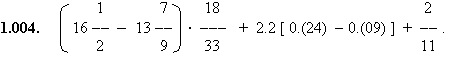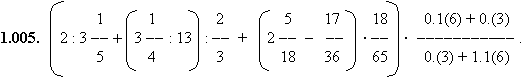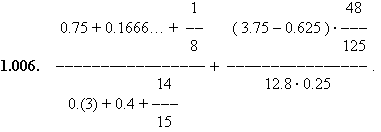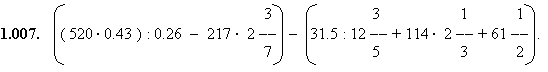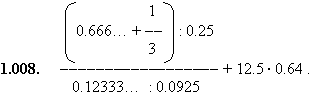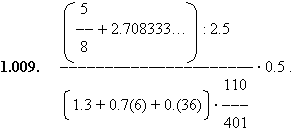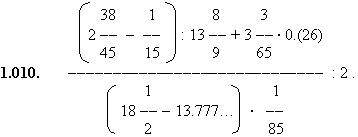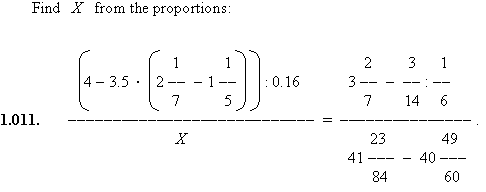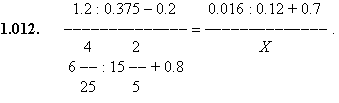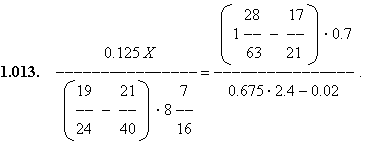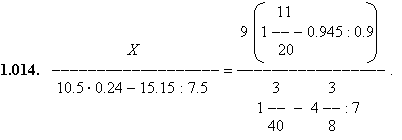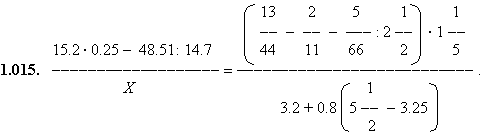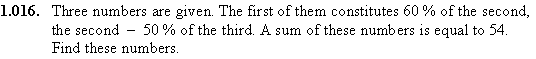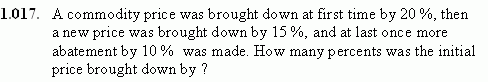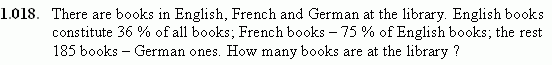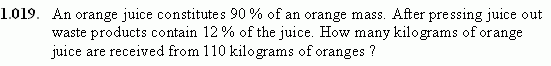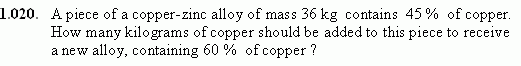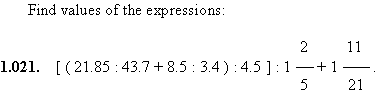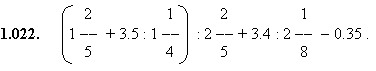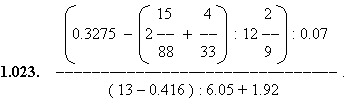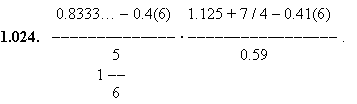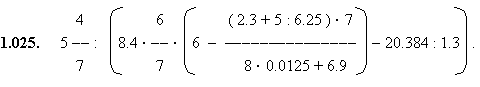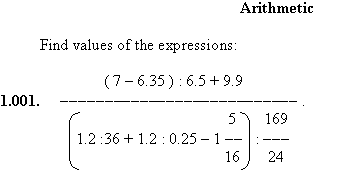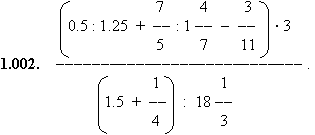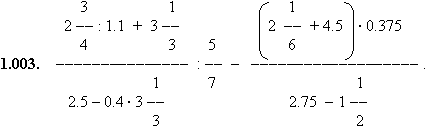The note. In examples no. no. 1.004 - 1.006, 1.008 - 1.010, 1.024 where decimal periodic fractions are, it is
possible to use for their transformation in simple fractions the following method. We’ll admit the fraction 0. (263)
is given. Let's designate it through x = 0.(263), and then multiply both parts of this equality by 1000 (more precisely,
by 10 n where n – a number of digits in the decimal fraction period), we’ll receive: 1000 x = 263.(263). From here
1000 x - 263 = x , or 999 x = 263, and finally, x = 263/999. Thus, 0.(263) = 263/999. The alternative method
of transformation of decimal periodic fraction in a simple fraction is given in solution of the example 1.004.